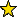# CodingTheory -- tools for Coding Theory

## Description

CodingTheory is a package designed to provide basic coding theory objects, and methods for computing the basic parameters of linear codes. Some of the implemented functions use commutative algebra techniques.

CodingTheory currently provides constructors for linear codes and evaluation codes, and a few methods for each.

## Contributors

Branden Stone generously contributed code or worked on our code at various Macaulay2 workshops.

## Authors

• Taylor Ball
• Eduardo Camps
• Henry Chimal-Dzul
• Delio Jaramillo-Velez
• Hiram H. Lopez
• Nathan Nichols
• Matthew Perkins
• Ivan Soprunov
• German Vera
• Gwyn Whieldon

## CertificationVersion 1.0 of this package was accepted for publication in volume 11 of The Journal of Software for Algebra and Geometry on 10 August 2021, in the article Coding theory package for Macaulay2. That version can be obtained from the journal or from the Macaulay2 source code repository.

## Version

This documentation describes version 1.0 of CodingTheory.

## Source code

The source code from which this documentation is derived is in the file CodingTheory.m2.

## Exports

• Types
• Functions and commands
• Methods
• "alphabet(LinearCode)" -- see alphabet -- elements of the base ring of a code
• "ambientSpace(LinearCode)" -- see ambientSpace -- recovers the ambient module of a code
• "bitflipDecode(Matrix,Vector,ZZ)" -- see bitflipDecode -- an experimental implementation of a message passing decoder
• "cartesianCode(Ring,List,List)" -- see cartesianCode -- Cartesian code
• "cartesianCode(Ring,List,Matrix)" -- see cartesianCode -- Cartesian code
• "cartesianCode(Ring,List,ZZ)" -- see cartesianCode -- Cartesian code
• "chooseStrat(LinearCode)" -- see chooseStrat -- Estimate the optimal strategy to compute the minimum weight of a linear code.
• "codewords(LinearCode)" -- see codewords -- codewords of the code
• "cyclicCode(GaloisField,RingElement,ZZ)" -- see cyclicCode -- cyclic codes
• "cyclicCode(GaloisField,ZZ,ZZ)" -- see cyclicCode -- cyclic codes
• "cyclicMatrix(GaloisField,List)" -- see cyclicMatrix -- cyclic matrix
• "cyclicMatrix(List)" -- see cyclicMatrix -- cyclic matrix
• dim(LinearCode) -- dimension of a linear code
• "dualCode(LinearCode)" -- see dualCode -- dual of a code
• "enumerateVectors(Ring,List)" -- see enumerateVectors -- a particular way to enumerate vectors over a finite field
• "evaluationCode(Ring,List,List)" -- see evaluationCode -- functions to construct evaluation codes over Galois fields
• "evaluationCode(Ring,List,Matrix)" -- see evaluationCode -- functions to construct evaluation codes over Galois fields
• "evCodeGraph(Ring,Matrix,List)" -- see evCodeGraph -- Reed–Muller-type code over a graph
• "field(LinearCode)" -- see field -- the field of a code
• "footPrint(ZZ,ZZ,Ideal)" -- see footPrint -- generalized footprint function of an ideal
• "generatorToParityCheck(Matrix)" -- see generatorToParityCheck -- parity check matrix of a linear code
• "genericCode(LinearCode)" -- see genericCode -- ambient space of a code
• "genMinDisIdeal(ZZ,ZZ,Ideal)" -- see genMinDisIdeal -- generalized minimum distance function of an ideal
• "getLRCencodingPolynomial(ZZ,ZZ,List,RingElement)" -- see getLRCencodingPolynomial -- encoding polynomial for an LRC code
• "hammingCode(ZZ,ZZ)" -- see hammingCode -- generates a Hamming code
• "hyp(ZZ,ZZ,Ideal)" -- see hyp -- hyp function of an ideal
• "informationRate(LinearCode)" -- see informationRate -- information rate of a code
• length(LinearCode) -- returns the length of a linear code
• "linearCode(GaloisField,List)" -- see linearCode -- functions to construct linear codes over Galois fields
• "linearCode(GaloisField,ZZ,List)" -- see linearCode -- functions to construct linear codes over Galois fields
• "linearCode(Matrix)" -- see linearCode -- functions to construct linear codes over Galois fields
• "linearCode(Module)" -- see linearCode -- functions to construct linear codes over Galois fields
• "linearCode(Module,List)" -- see linearCode -- functions to construct linear codes over Galois fields
• "linearCode(ZZ,ZZ,ZZ,List)" -- see linearCode -- functions to construct linear codes over Galois fields
• LinearCode == LinearCode -- determines if two linear codes are equal
• "locallyRecoverableCode(List,List,RingElement)" -- see locallyRecoverableCode -- constructs a locally recoverable code (LRC)
• "messages(LinearCode)" -- see messages -- set of messages to be encoded by a code
• "minimumWeight(LinearCode)" -- see minimumWeight -- computes the minimum weight of a linear code
• "orderCode(Ideal,List,List,ZZ)" -- see orderCode -- computes an order code for a given weight
• "orderCode(Ideal,List,ZZ)" -- see orderCode -- computes an order code for a given weight
• "orderCode(Ring,List,List,ZZ)" -- see orderCode -- computes an order code for a given weight
• "parityCheckToGenerator(Matrix)" -- see parityCheckToGenerator -- generator matrix given a parity check matrix
• "quasiCyclicCode(GaloisField,List)" -- see quasiCyclicCode -- constructs a quasi-cyclic code
• "quasiCyclicCode(List)" -- see quasiCyclicCode -- constructs a quasi-cyclic code
• "randLDPC(ZZ,ZZ,RR,ZZ)" -- see randLDPC -- low density parity check matrix
• "randNoRepeats(ZZ,ZZ)" -- see randNoRepeats -- list of random integers from a specified range with no repetitions
• "randomCode(GaloisField,ZZ,ZZ)" -- see randomCode -- constructs a random linear code over a finite field
• "randomCode(QuotientRing,ZZ,ZZ)" -- see randomCode -- constructs a random linear code over a finite field
• "reducedMatrix(Matrix)" -- see reducedMatrix -- reduced matrix
• "reedMullerCode(ZZ,ZZ,ZZ)" -- see reedMullerCode -- constructs the Reed-Muller code
• "reedSolomonCode(Ring,List,ZZ)" -- see reedSolomonCode -- constructs the Reed-Solomon code
• "repetitionCode(GaloisField,ZZ)" -- see repetitionCode -- repetition code
• ring(LinearCode) -- the ring of a code
• "shorten(LinearCode,List)" -- see shorten -- shortens a code
• "shorten(LinearCode,ZZ)" -- see shorten -- shortens a code
• "shortestPath(Digraph,Thing,List)" -- see shortestPath -- shorthest path in a digraph
• size(LinearCode) -- gives the number of codewords in a linear code
• "syndromeDecode(LinearCode,Matrix,ZZ)" -- see syndromeDecode -- syndrome decoding on a code
• "tannerGraph(Matrix)" -- see tannerGraph -- outputs the tanner graph associated with the given parity check matrix
• "toricCode(Ring,Matrix)" -- see toricCode -- a toric code construction
• toString(LinearCode) -- string with the vectors of a generator matrix of a code
• "universeCode(GaloisField,ZZ)" -- see universeCode -- linear code $\mathtt{F}^\mathtt{n}$
• "vasconcelosDegree(ZZ,ZZ,Ideal)" -- see vasconcelosDegree -- Vasconcelos function of an ideal
• "vectorSpace(LinearCode)" -- see vectorSpace -- vector space of a code
• "vNumber(Ideal)" -- see vNumber -- the v-number of a graded ideal
• "weight(BasicList)" -- see weight -- Hamming weight of a list
• "zeroCode(GaloisField,ZZ)" -- see zeroCode -- zero code
• "zeroSumCode(GaloisField,ZZ)" -- see zeroSumCode -- linear code in which the entries of each codeword add up zero
• Symbols

## For the programmer

The object CodingTheory is .